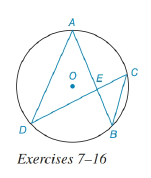Chapter 6.3, Problem 12EElementary Geometry For College St...

7th Edition
Alexander + 2 others
ISBN: 9781337614085

Solutions

Chapter
SectionElementary Geometry For College St...

7th Edition
Alexander + 2 others
ISBN: 9781337614085
Textbook Problem

In the figure for Exercises 7 to 16, O is the center of the circle. See Theorem 6.3.5.Given: A D = 10 , B C = 4 , A E = 7 Find: EC.To determine

To find:

The value of EC.

Explanation

The diagrammatic representation is given below,

Theorem:

1. If two chords intersect within a circle, then the product of the lengths of the segments (parts) of one chord is equal to the product of the lengths of the segments of the other chord. Now compare the triangles ΔAED,ΔCEB

2. If two inscribed angles intercept the same arc, then these angles congruent. By using the above theorem mADE=mCBE

Vertical angles are congruent to each other. The angles that are opposite to each other are vertical angles.

By suing the vertical angle theorem to get the following,

mAED=mCEB

Since by Angle rule we conclude that

m

Still sussing out bartleby?

Check out a sample textbook solution.

See a sample solution

The Solution to Your Study Problems

Bartleby provides explanations to thousands of textbook problems written by our experts, many with advanced degrees!

Get Started

Let f be the function defined by f(t)=2t2t1. Find f(2), f(a), f(x + 1), and f(x 1).

Applied Calculus for the Managerial, Life, and Social Sciences: A Brief Approach

Evaluate the iterated integral: 0204y224y2dxdy

Calculus: Early Transcendental Functions

Polynomial Inequalities Solve the inequality. 8. 2x3 18x x2 9

Precalculus: Mathematics for Calculus (Standalone Book)

Evaluate the integral by making the given substitution. sin2cosd,u=sin

Single Variable Calculus: Early Transcendentals, Volume I

Convert the expressions in Exercises 8596 radical form. y7/4

Finite Mathematics and Applied Calculus (MindTap Course List)

Prove the identity. 56. tanx+tany=sin(x+y)cosxcosy

Single Variable Calculus: Early Transcendentals

True or False: If f(x) 0 for x c and f(x) 0 for x c, then c is a point of inflection.

Study Guide for Stewart's Single Variable Calculus: Early Transcendentals, 8th

True or False: , for –π ≤ t ≤ π, is smooth.

Study Guide for Stewart's Multivariable Calculus, 8th